Composing & Decomposing Numbers (1-10) | Grades K, 1, & 2
1%
It was processed successfully!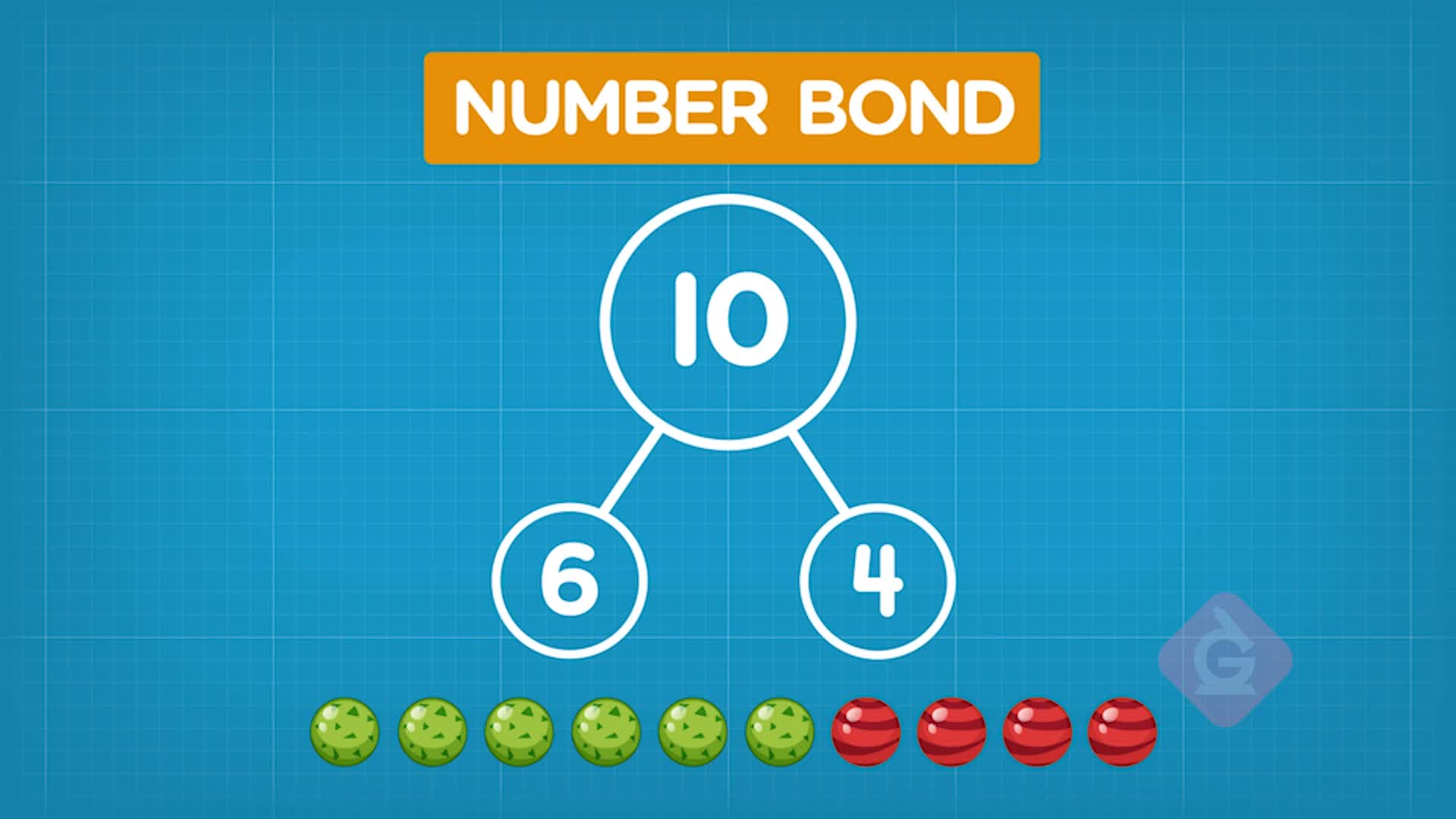WHAT ARE COMPOSING & DECOMPOSING NUMBERS (1-10)?

You will learn that whole numbers can be put together to compose a greater number. Whole numbers can also be broken apart to decompose into two lesser numbers!

To better understand composing & decomposing numbers…

WHAT ARE COMPOSING & DECOMPOSING NUMBERS (1-10)?. You will learn that whole numbers can be put together to compose a greater number. Whole numbers can also be broken apart to decompose into two lesser numbers! To better understand composing & decomposing numbers…

## LET’S BREAK IT DOWN!

### Decompose a number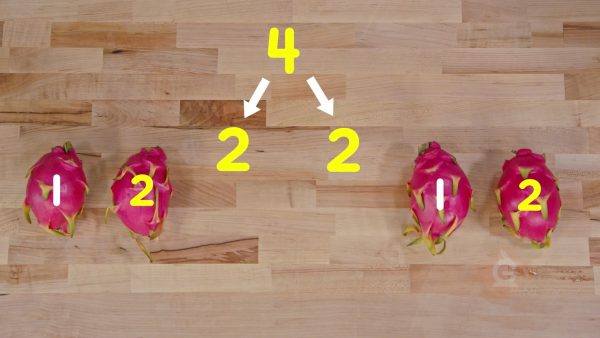Decomposing a number means taking a whole number and breaking it into two smaller parts. To decompose the number 5, you can break it into smaller parts of 4 and 1, or 3 and 2.

Decompose a number Decomposing a number means taking a whole number and breaking it into two smaller parts. To decompose the number 5, you can break it into smaller parts of 4 and 1, or 3 and 2.

### Use a number bond to show parts.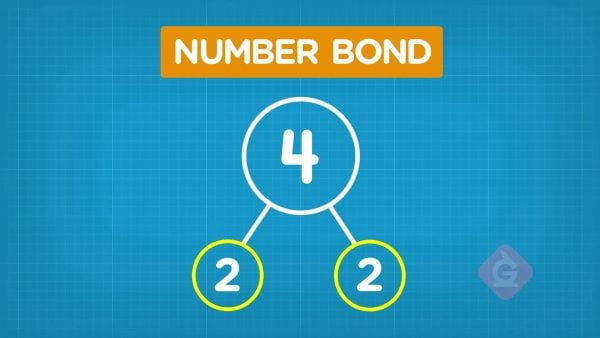A number bond is a tool you can use to show parts of a whole. A number bond has one circle on top and two circles on the bottom. The top number shows the whole. The bottom numbers show the parts.

Use a number bond to show parts. A number bond is a tool you can use to show parts of a whole. A number bond has one circle on top and two circles on the bottom. The top number shows the whole. The bottom numbers show the parts.

### Decompose 8 using a number bond.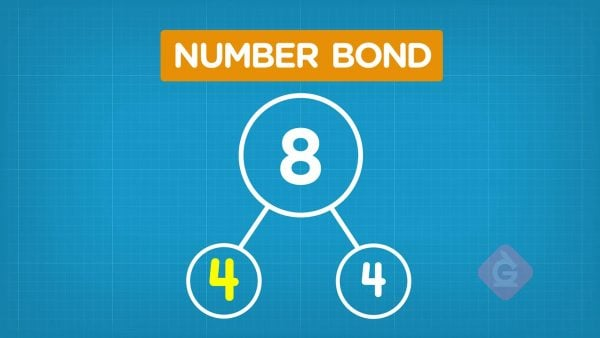To show 8 in a number bond, put 8 in the top circle. Then the parts go in the lower circles. 2 and 6 make 8. 2 and 6 can go in the lower circles.

Decompose 8 using a number bond. To show 8 in a number bond, put 8 in the top circle. Then the parts go in the lower circles. 2 and 6 make 8. 2 and 6 can go in the lower circles.

### Compose a number.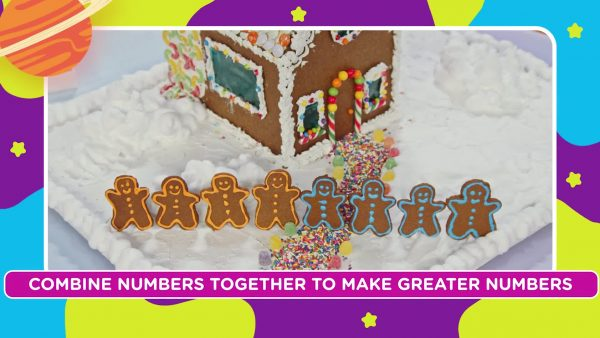Composing a number means taking two lesser numbers and combining them to make a greater whole number. A group of 4 and a group of 2 make 6.

Compose a number. Composing a number means taking two lesser numbers and combining them to make a greater whole number. A group of 4 and a group of 2 make 6.

### Compose 9 using a number bond.9 goes in the top circle. 6 and 3 make 9 so the numbers 6 and 3 can go in the lower circles. You can say 6 and 3 make 9 OR 3 and 6 make 9.

Compose 9 using a number bond. 9 goes in the top circle. 6 and 3 make 9 so the numbers 6 and 3 can go in the lower circles. You can say 6 and 3 make 9 OR 3 and 6 make 9.

## COMPOSING & DECOMPOSING NUMBERS (1-10) VOCABULARY

Decomposing numbers
Breaking a number into two smaller parts.
Composing numbers
Combining two parts of a whole.
Number Bond
A diagram that shows a whole and two parts.
Total
The result of counting.
Count
The find how many items in a group.
Numbers
Symbols used to represent how many.

## COMPOSING & DECOMPOSING NUMBERS (1-10) DISCUSSION QUESTIONS

### There are 8 dragon fruits. Gavin has 5. How many does Amiyah have?

5 and 3 make 8, so Amiyah has 3 dragon fruit.

### What does a number bond show us?

The parts and the whole.

### [Draw a number bond showing 9 broken into 4 and an unknown amount.] What part is missing from this number bond? How do you know?

5. 4 and 5 make 9.

### [Draw a number bond showing an unknown number broken into 3 and 7.] What whole is missing from this number bond? How do you know?

3 and 7 make 10, so 10 is the missing number.

2 and 4 make 6.
X

## Success

We’ve sent you an email with instructions how to reset your password.
Ok

## Choose Your Free Trial Period### 7 DaysContinue to Lessons### 90 DaysGet 90 days free by inviting other teachers to try it too.

Share with Teachers

## Get 90 Days Free

### By inviting 4 other teachers to try it too.

4 required

Skip, I will use a 7 days free trial

## Thank You!

Enjoy your free 90 days trial